Case Based Questions (MCQ)

Chapter 13 Class 12 Probability
Serial order wise

## Based on the above information answer the  following: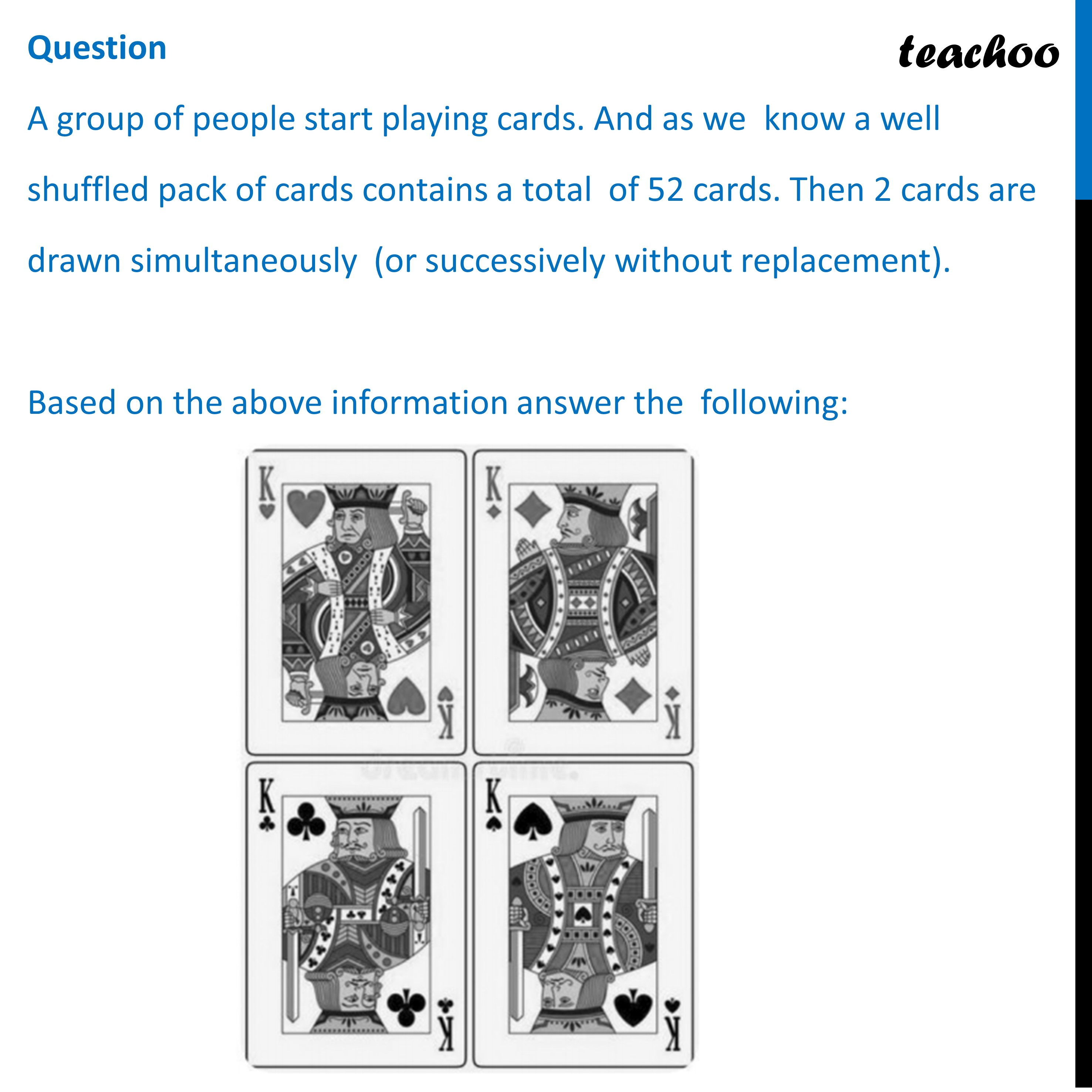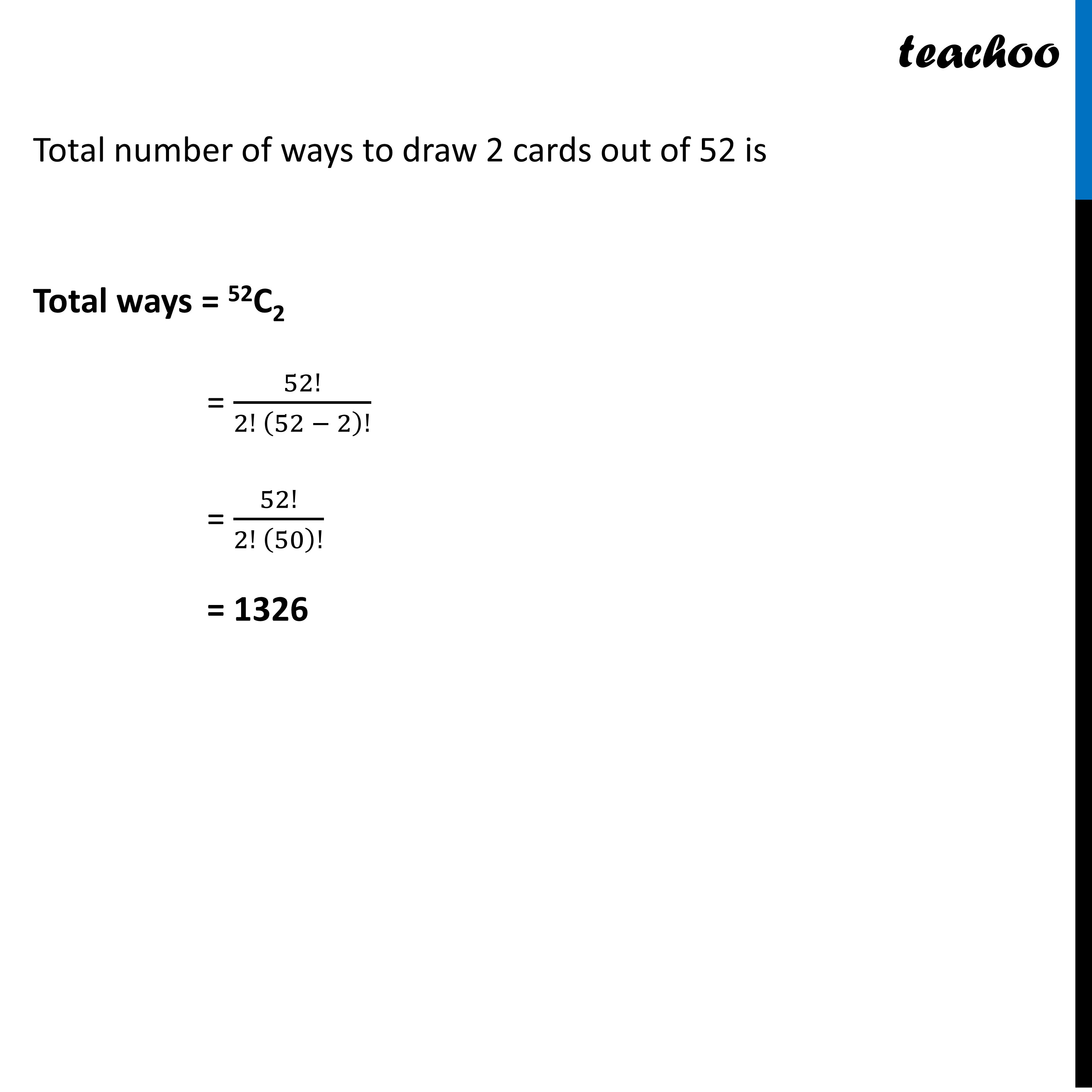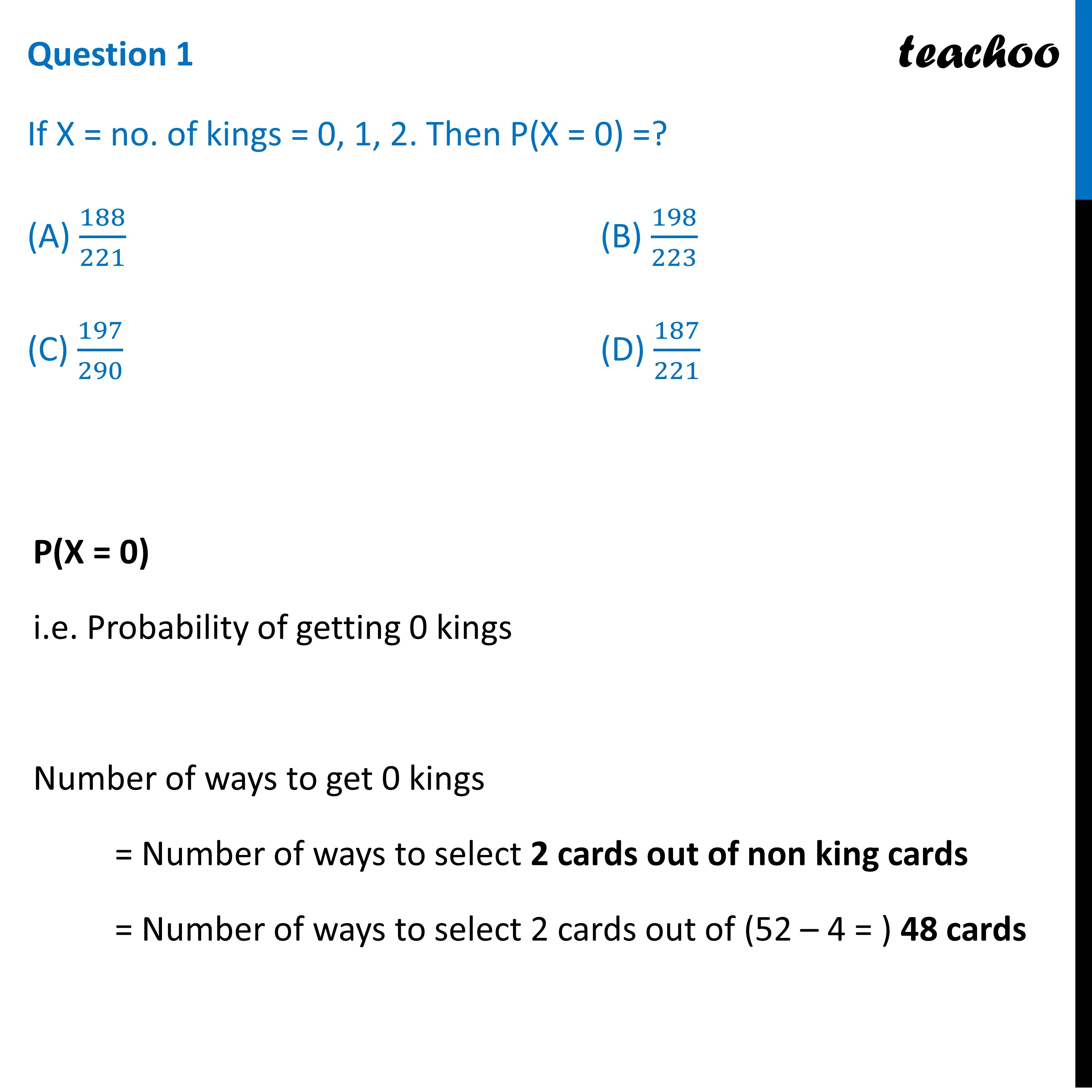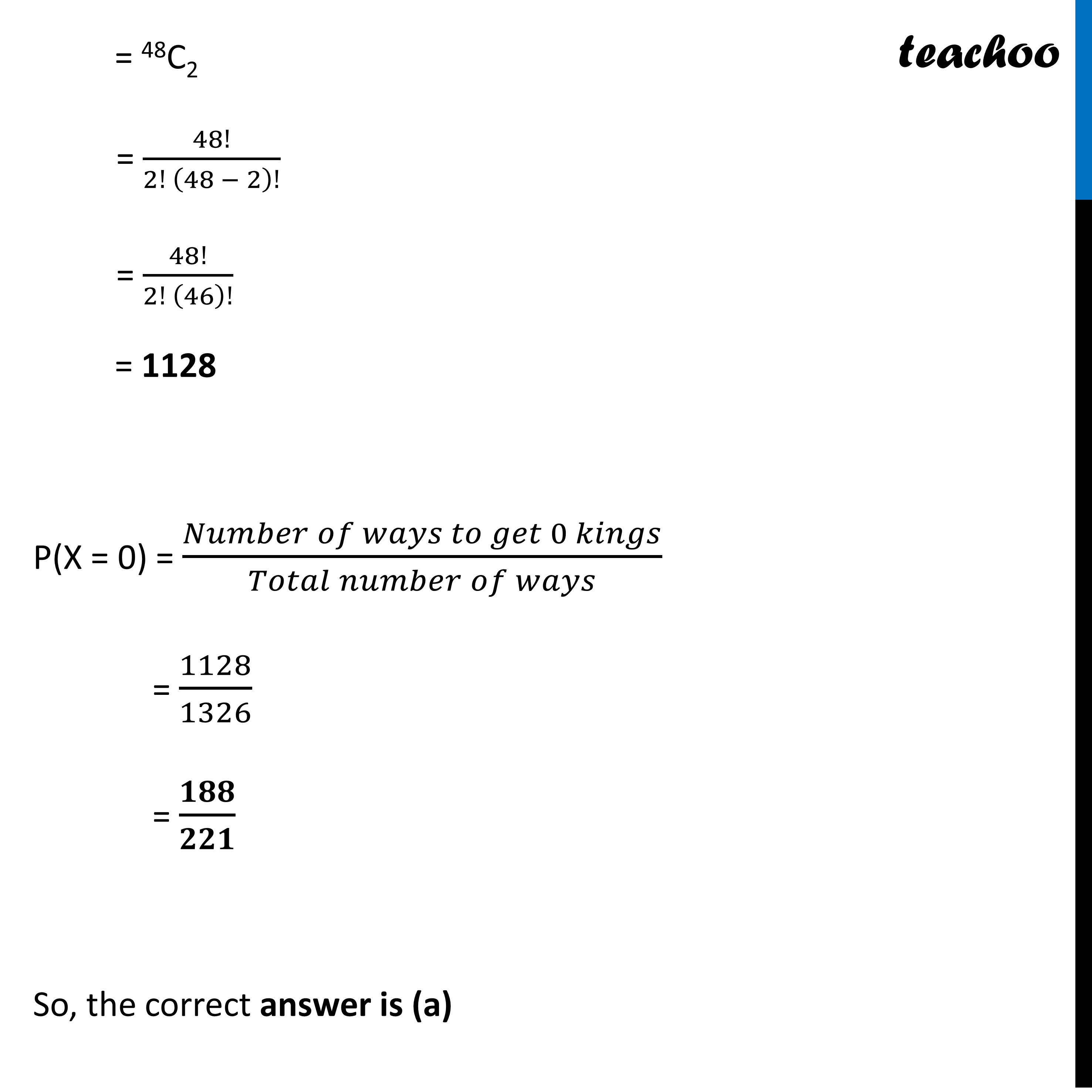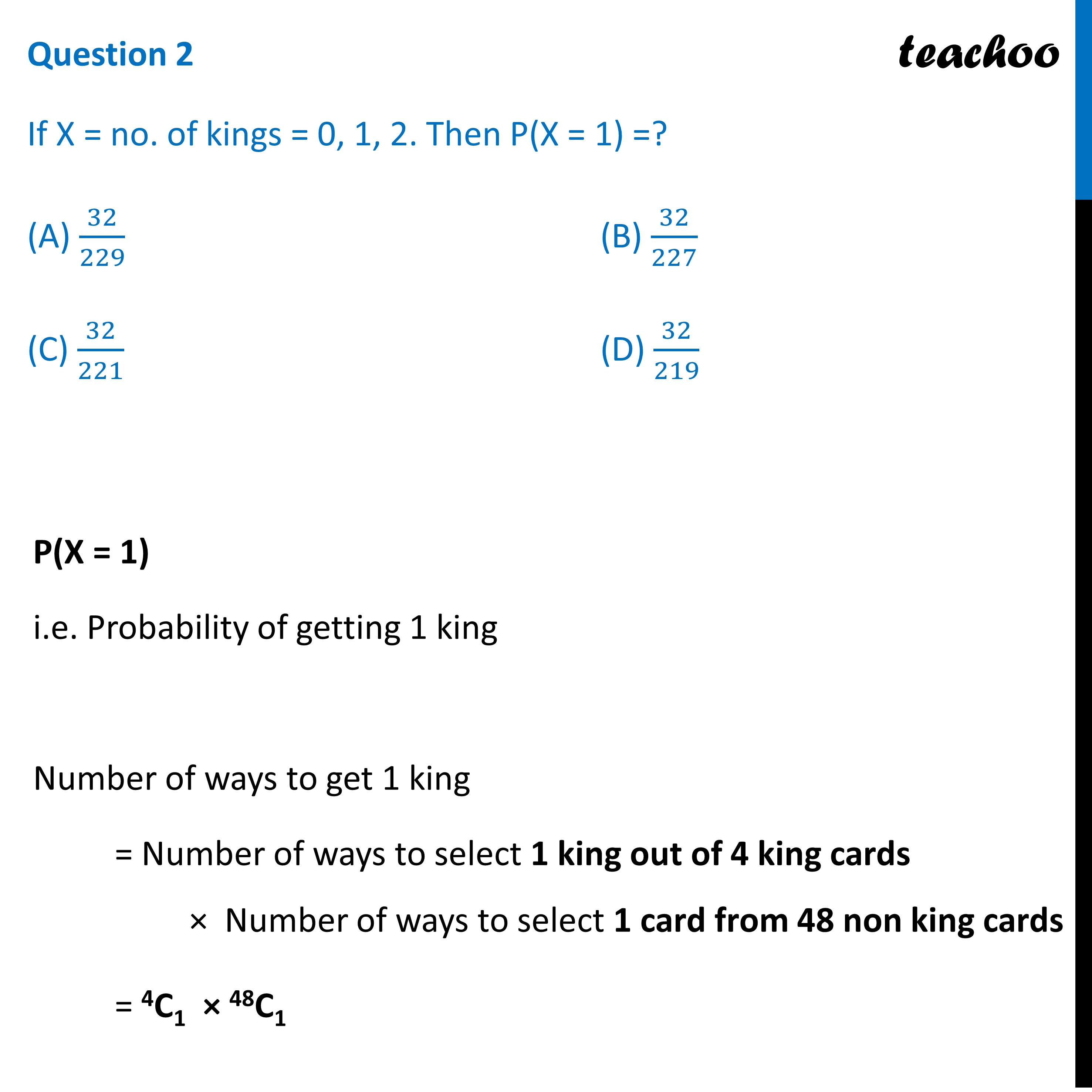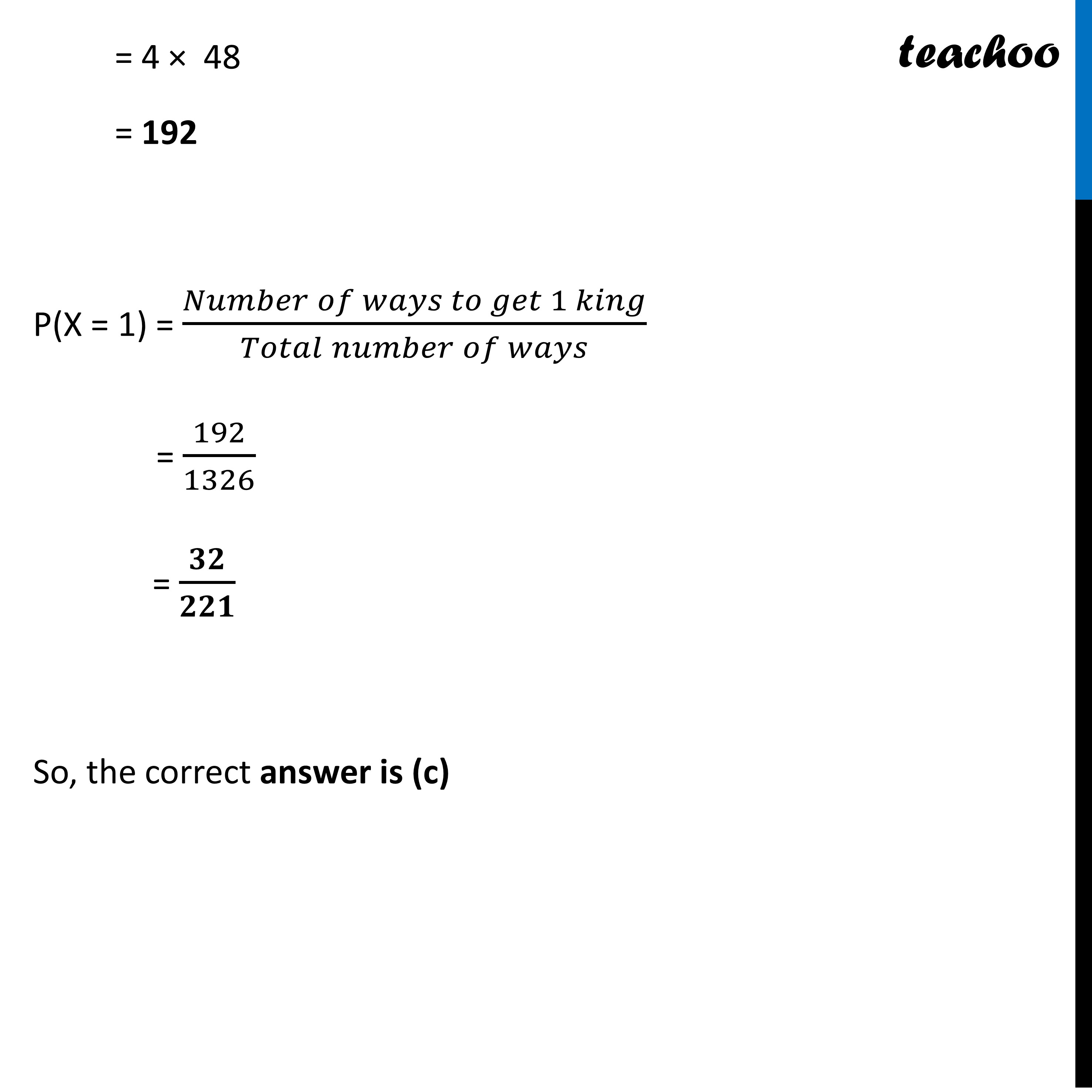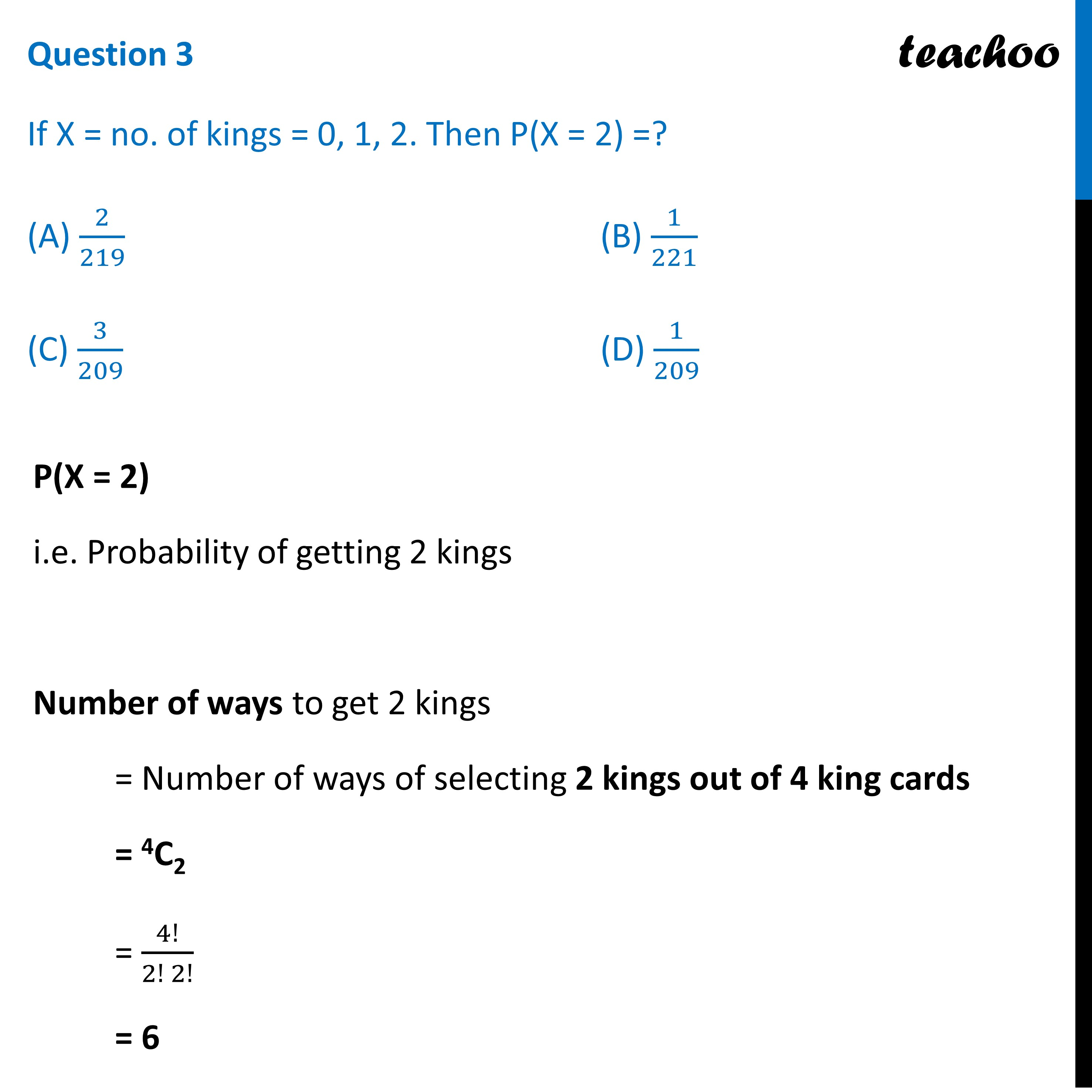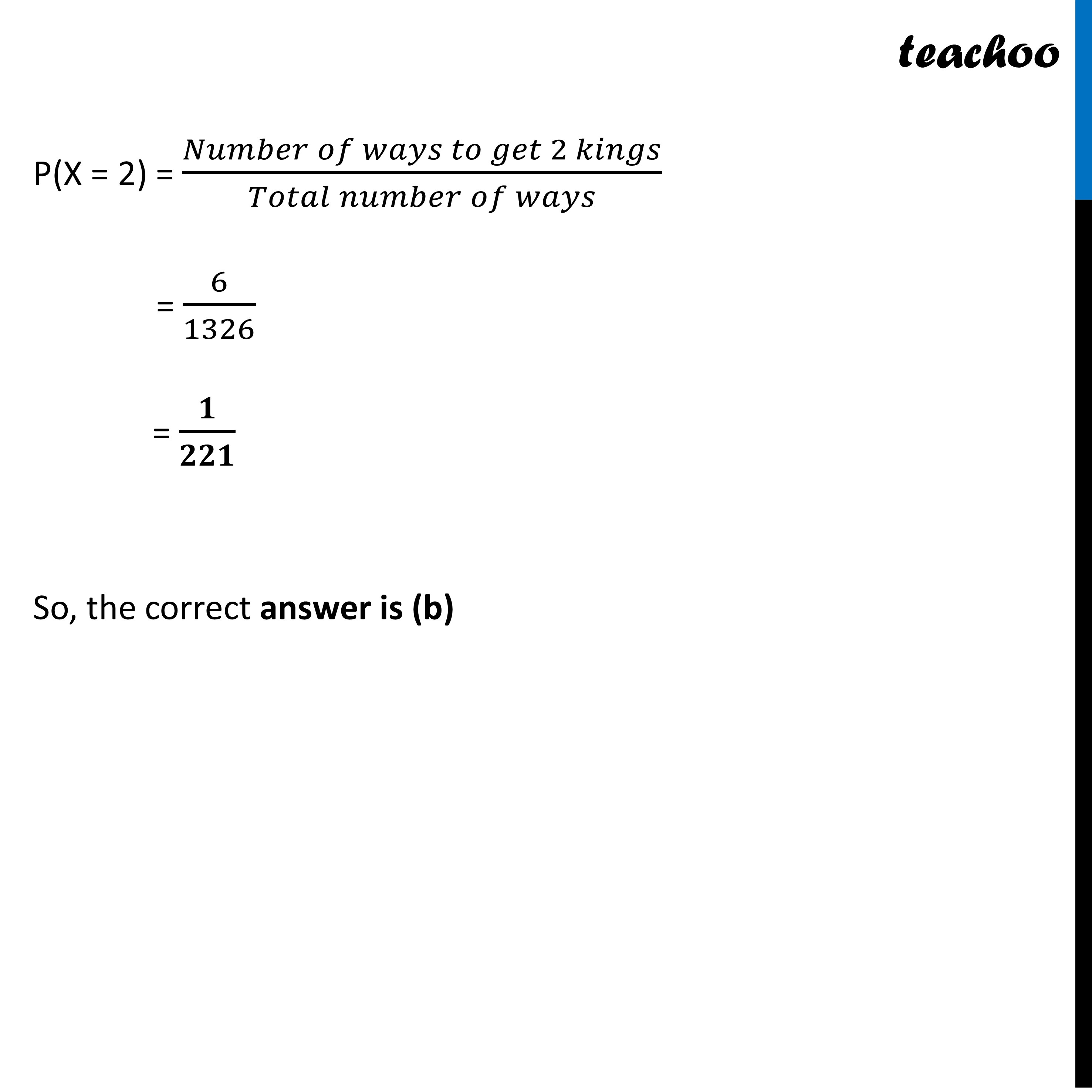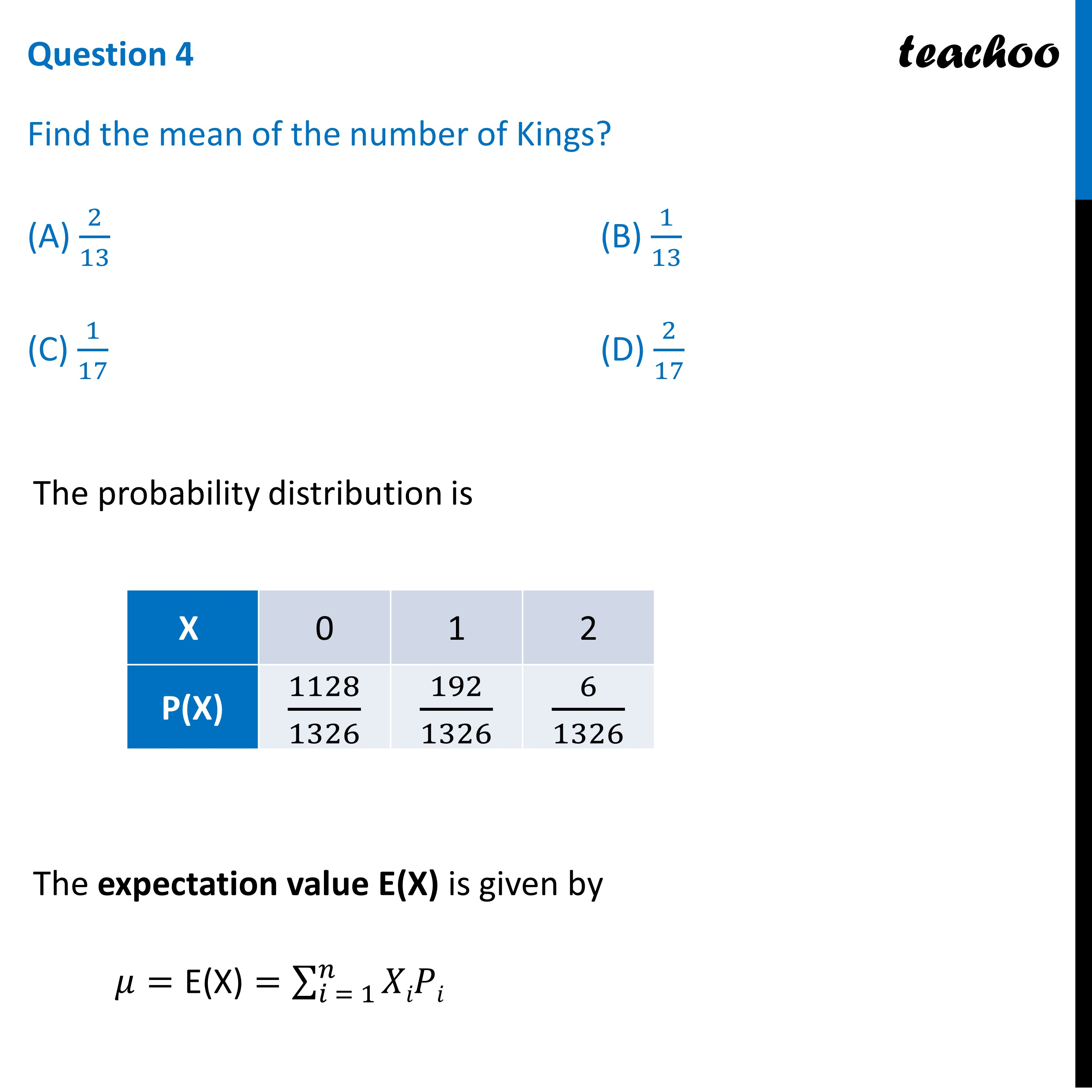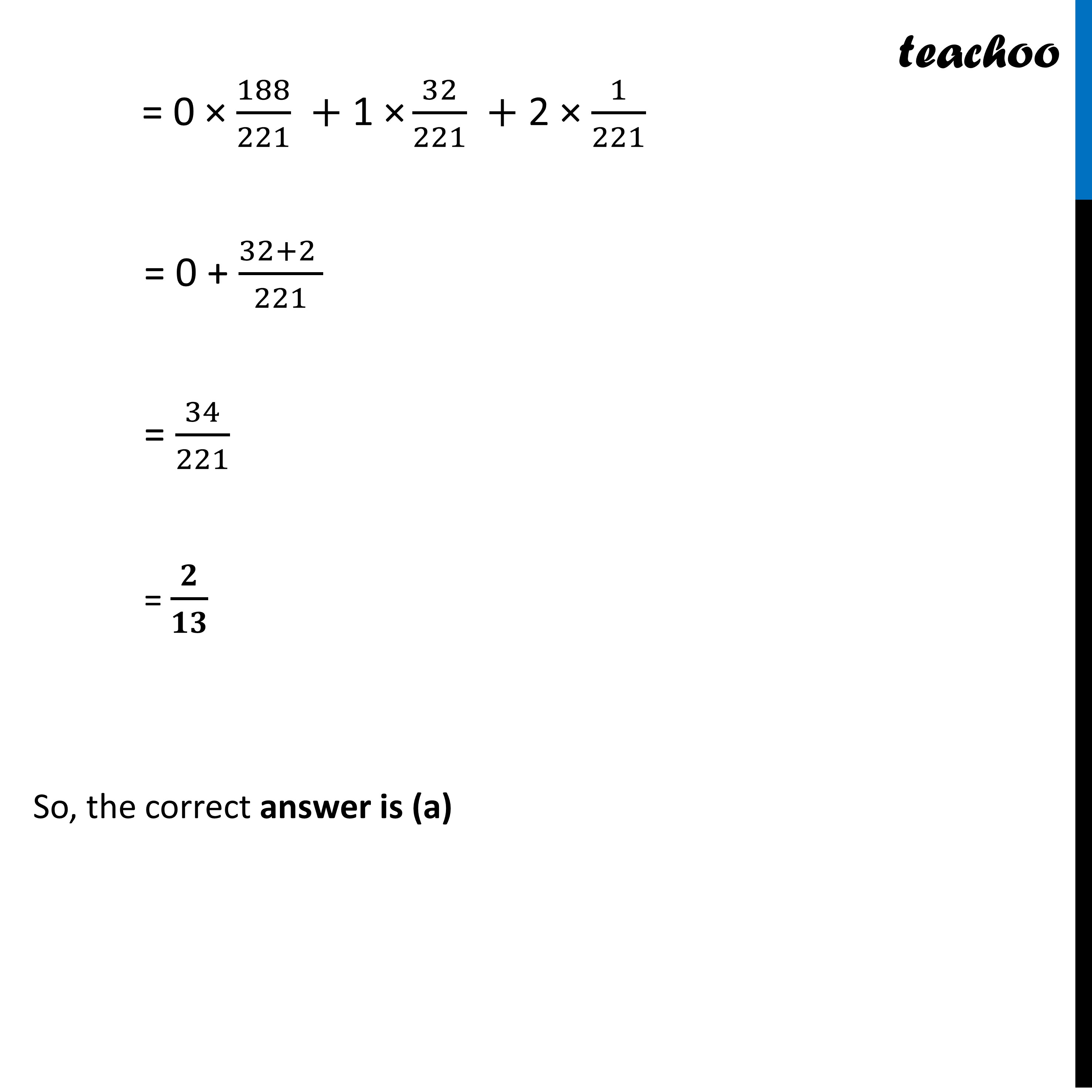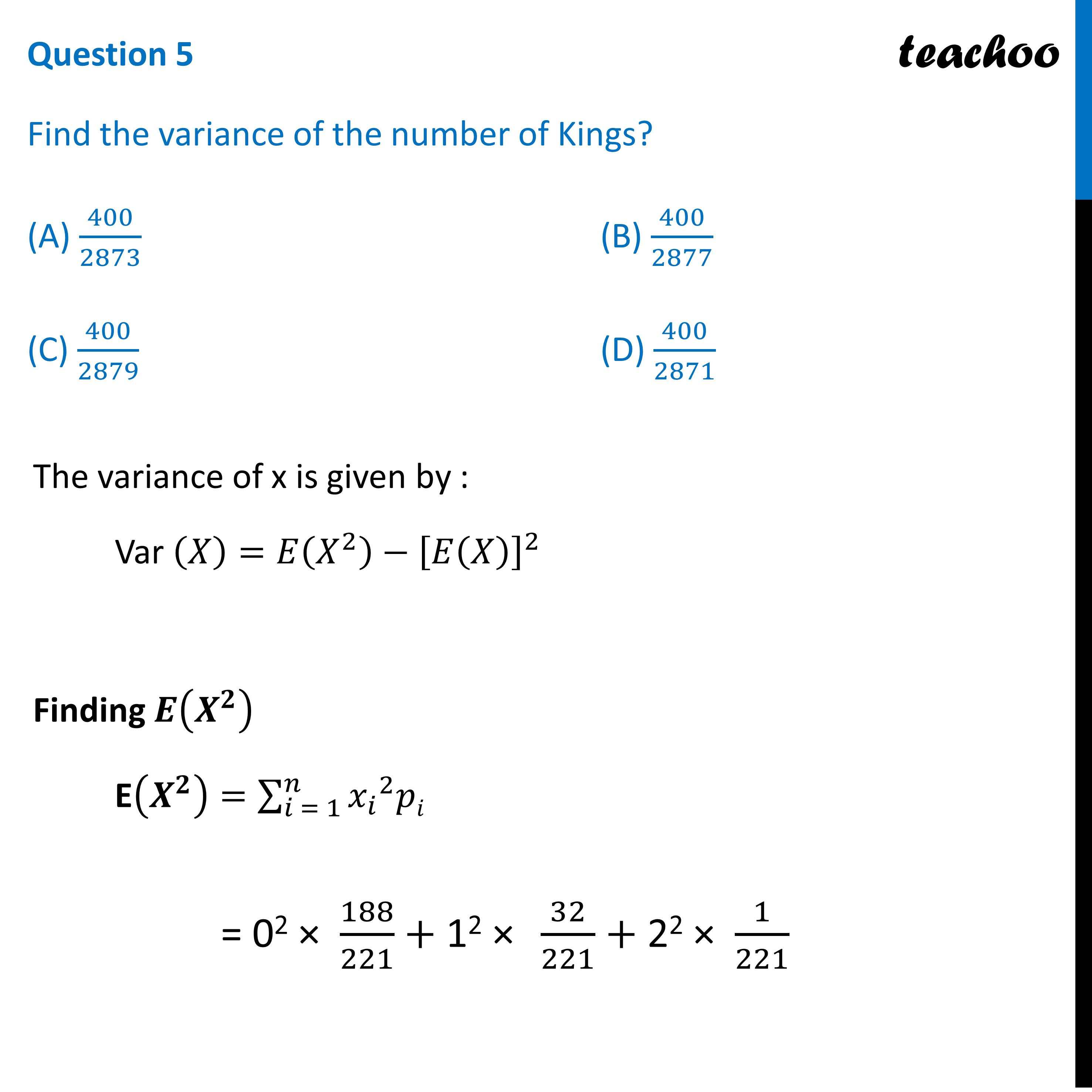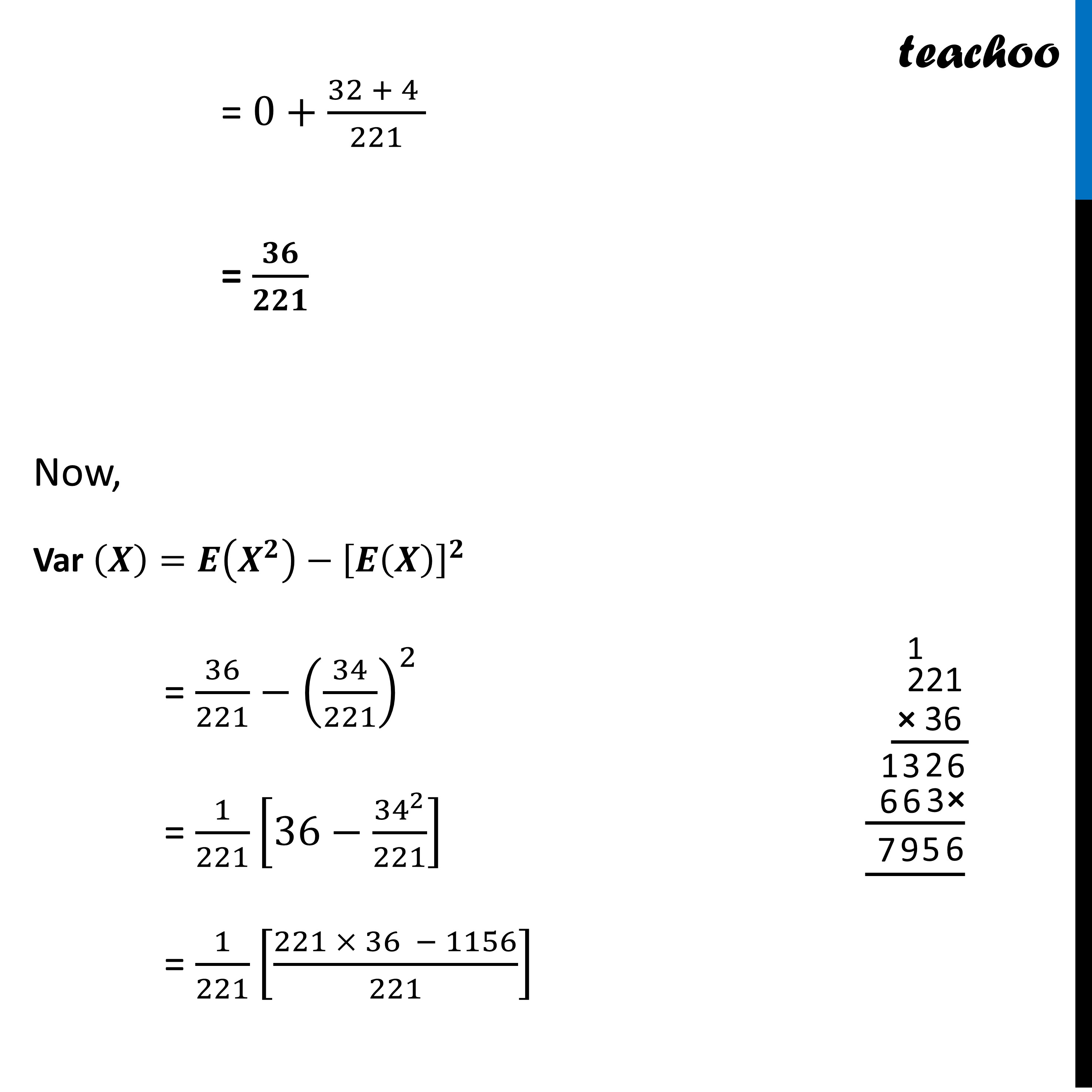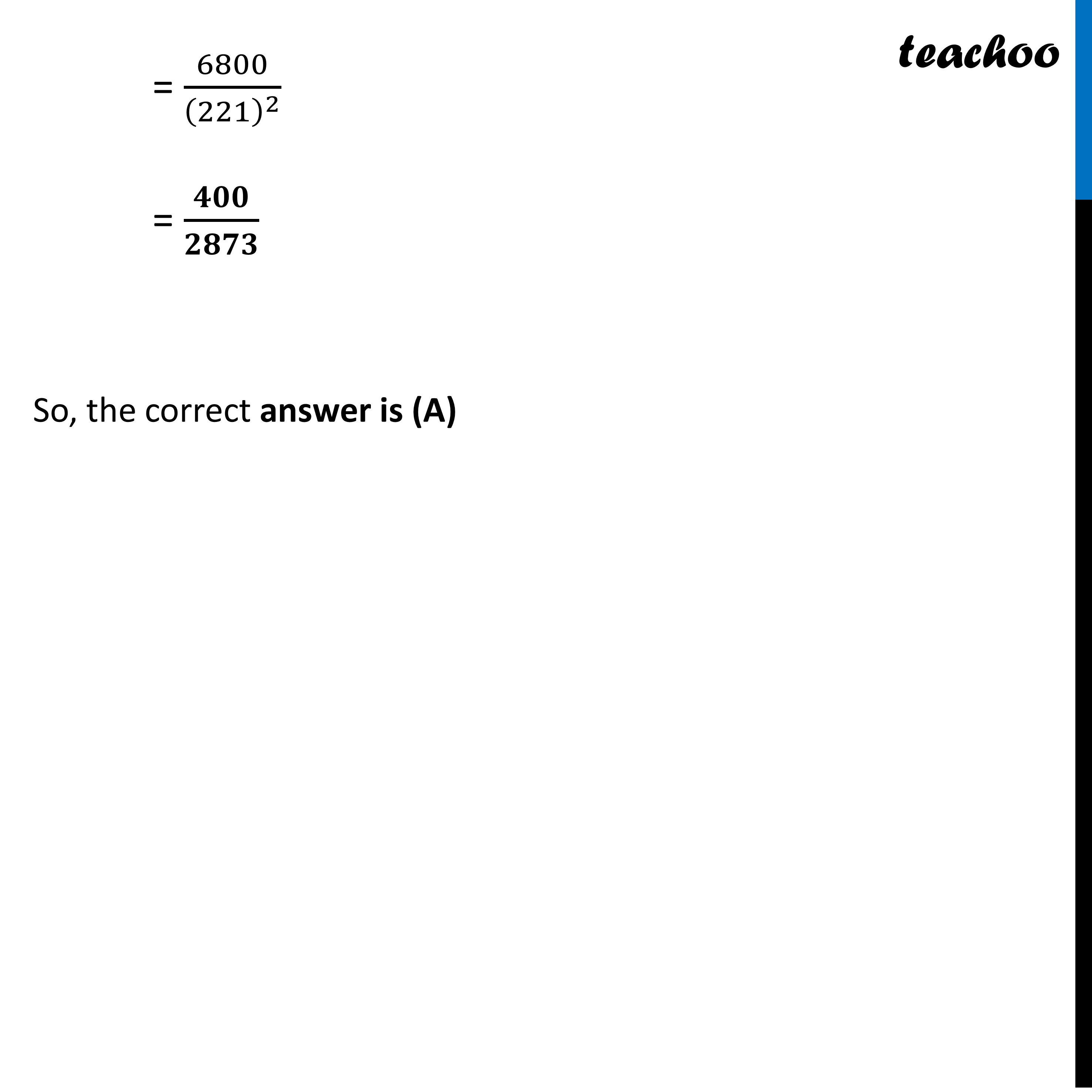Learn in your speed, with individual attention - Teachoo Maths 1-on-1 Class

### Transcript

Question A group of people start playing cards. And as we know a well shuffled pack of cards contains a total of 52 cards. Then 2 cards are drawn simultaneously (or successively without replacement). Based on the above information answer the following: Total number of ways to draw 2 cards out of 52 is Total ways = 52C2 = 52!/(2! (52 − 2)!) = 52!/(2! (50)!) = 1326 Question 1 If X = no. of kings = 0, 1, 2. Then P(X = 0) =? (A) 188/221 (B) 198/223 (C) 197/290 (D) 187/221 P(X = 0) i.e. Probability of getting 0 kings Number of ways to get 0 kings = Number of ways to select 2 cards out of non king cards = Number of ways to select 2 cards out of (52 – 4 = ) 48 cards = 48C2 = 48!/(2! (48 − 2)!) = 48!/(2! (46)!) = 1128 P(X = 0) = (𝑁𝑢𝑚𝑏𝑒𝑟 𝑜𝑓 𝑤𝑎𝑦𝑠 𝑡𝑜 𝑔𝑒𝑡 0 𝑘𝑖𝑛𝑔𝑠)/(𝑇𝑜𝑡𝑎𝑙 𝑛𝑢𝑚𝑏𝑒𝑟 𝑜𝑓 𝑤𝑎𝑦𝑠) = 1128/1326 = 𝟏𝟖𝟖/𝟐𝟐𝟏 So, the correct answer is (a) Question 2 If X = no. of kings = 0, 1, 2. Then P(X = 1) =? (A) 32/229 (B) 32/227 (C) 32/221 (D) 32/219 P(X = 1) i.e. Probability of getting 1 king Number of ways to get 1 king = Number of ways to select 1 king out of 4 king cards × Number of ways to select 1 card from 48 non king cards = 4C1 × 48C1 = 4 × 48 = 192 P(X = 1) = (𝑁𝑢𝑚𝑏𝑒𝑟 𝑜𝑓 𝑤𝑎𝑦𝑠 𝑡𝑜 𝑔𝑒𝑡 1 𝑘𝑖𝑛𝑔)/(𝑇𝑜𝑡𝑎𝑙 𝑛𝑢𝑚𝑏𝑒𝑟 𝑜𝑓 𝑤𝑎𝑦𝑠) = 192/1326 = 𝟑𝟐/𝟐𝟐𝟏 So, the correct answer is (c) Question 3 If X = no. of kings = 0, 1, 2. Then P(X = 2) =? (A) 2/219 (B) 1/221 (C) 3/209 (D) 1/209 P(X = 2) i.e. Probability of getting 2 kings Number of ways to get 2 kings = Number of ways of selecting 2 kings out of 4 king cards = 4C2 = 4!/(2! 2!) = 6 P(X = 2) = (𝑁𝑢𝑚𝑏𝑒𝑟 𝑜𝑓 𝑤𝑎𝑦𝑠 𝑡𝑜 𝑔𝑒𝑡 2 𝑘𝑖𝑛𝑔𝑠)/(𝑇𝑜𝑡𝑎𝑙 𝑛𝑢𝑚𝑏𝑒𝑟 𝑜𝑓 𝑤𝑎𝑦𝑠) = 6/1326 = 𝟏/𝟐𝟐𝟏 So, the correct answer is (b) Question 4 Find the mean of the number of Kings? (A) 2/13 (B) 1/13 (C) 1/17 (D) 2/17 The probability distribution is The expectation value E(X) is given by 𝜇="E(X)"=∑2_(𝑖 = 1)^𝑛▒𝑋𝑖𝑃𝑖 = 0 × 188/221 +"1 ×" 32/221 + 2 × 1/221 = 0 + (32+2 )/221 = 34/221 = 𝟐/𝟏𝟑 So, the correct answer is (a) Question 5 Find the variance of the number of Kings? (A) 400/2873 (B) 400/2877 (C) 400/2879 (D) 400/2871 The variance of x is given by : Var (𝑋)=𝐸(𝑋^2 )−[𝐸(𝑋)]^2 Finding 𝑬(𝑿^𝟐 ) E(𝑿^𝟐 )=∑2_(𝑖 = 1)^𝑛▒〖〖𝑥_𝑖〗^2 𝑝𝑖〗 = 02 × 188/221+"12 × " 32/221+ 22 × 1/221= 0+(32 + 4 )/221 = 𝟑𝟔/𝟐𝟐𝟏 Now, Var (𝑿)=𝑬(𝑿^𝟐 )−[𝑬(𝑿)]^𝟐 = 36/221−(34/221)^2 = 1/221 [36−〖34〗^2/221] = 1/221 [(221 × 36 − 1156)/221] = 6800/(221)^2 = 𝟒𝟎𝟎/𝟐𝟖𝟕𝟑 So, the correct answer is (A)